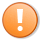# Quick-tip 60 | Calculate the linear regression of a Hull moving average

_Quick-tip 60 is a TradeStation EasyLanguage tutorial for TradeStation 10 that demonstrates how to calculate the linear regression of a Hull moving average and draws a line on the chart for each new bar that forms. It is based on tutorial 48 which was modified to calculate and draw a linear regression of a Hull moving average rather than the closing price of the bar.This content is for members only.